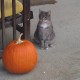# Prismatic puzzle

This week’s Riddler Classic is simple to state, but tricky to figure out:

What whole number dimensions can rectangular prisms have so that their volume (in cubic units) is the same as their surface area (in square units)?

Here is my solution:
[Show Solution]

## 5 thoughts on “Prismatic puzzle”

1.Ben says:

You can use ordering to show that c is at least 3, this leaves the most space for b, a. If c is 3 then b is at least 7, again maximizing a. If c is 3 and b is 7 then a is 42.

Fun fact is that in higher dimensions you can keep going along this route to get that if the max side for dimension d is m_d then m_{d+1}=m_d*(m_d+1)

Unfortunately this becomes really big really fast

2.Dave Moran says:

This is really a nice solution. I got stumped by this one and thought it could be solved only by coding.

3.Julian says:

As someone who enjoys solving The Riddler as well, I always look forward to the way you approach these problems, it’s very cool! I just brute forced it in R like so: https://julianegerez.github.io/blog/2019/12/19/The-Riddler-Prismatic-Puzzle but I was left thinking about how to approach this more rigorously, so thanks for that.

This doesn’t use ordering, but https://www.wolframalpha.com/input/?i=integer+solutions+to+abc+%3D+2%28ab%2Bbc%2Bca%29 tells me that: a>=3 and b>(2 a)/(a – 2) and c = (2 a b)/(a (b – 2) – 2 b). Does that provide any additional insight?

4.Ravi Fernando says:

Divide your first equation by 2abc and it reduces to finding three unit fractions whose sum is 1/2. This feels much simpler, although the casework will be the same. The same idea works in higher dimensions: the answer is the number of ways to represent 1/2 as a sum of n unit fractions. Equivalently, it’s the number of ways to represent 1 as a sum of n+1 unit fractions, where one of the fractions must be 1/2. This implies an upper bound on the dimensions in terms of the Sylvester sequence.

It’s very surprising that the answer is not known for 7. According to https://oeis.org/A002966, it was calculated in 2004 that there are 159330691 ways to express 1 as the sum of 8 unit fractions, and we just need to know how many of those contain the fraction 1/2. In general, the answer to this problem will be at most A002966(n+1) (set one of the n+1 fractions equal to 1/2), but at least A002966(n) (double all the denominators).

5.a villani says:

Hi, These 10 solutions are the same as those found by tesselation/tiling equations for 3 polygons of sides n. 4 such combinations tile the plane (e.g. 6,6,6, three hexagons, 4,8,8 two octagons and a square) while 6 are “forbidden”, including my favorite, the 42-gon! Can someone cleverer than I am explain why this appears to be the same math problem? Great job with this proof!
https://blogs.ams.org/visualinsight/2015/02/01/pentagon-decagon-packing/